The Nature of Light

# 2 The Propagation of Light

### Learning Objectives

By the end of this section, you will be able to:

• Determine the index of refraction, given the speed of light in a medium
• List the ways in which light travels from a source to another location

The speed of light in a vacuum c is one of the fundamental constants of physics. As you will see when you reach Relativity, it is a central concept in Einstein’s theory of relativity. As the accuracy of the measurements of the speed of light improved, it was found that different observers, even those moving at large velocities with respect to each other, measure the same value for the speed of light. However, the speed of light does vary in a precise manner with the material it traverses. These facts have far-reaching implications, as we will see in later chapters.

### The Speed of Light: Early Measurements

The first measurement of the speed of light was made by the Danish astronomer Ole Roemer (1644–1710) in 1675. He studied the orbit of Io, one of the four large moons of Jupiter, and found that it had a period of revolution of 42.5 h around Jupiter. He also discovered that this value fluctuated by a few seconds, depending on the position of Earth in its orbit around the Sun. Roemer realized that this fluctuation was due to the finite speed of light and could be used to determine c.

Roemer found the period of revolution of Io by measuring the time interval between successive eclipses by Jupiter. (Figure)(a) shows the planetary configurations when such a measurement is made from Earth in the part of its orbit where it is receding from Jupiter. When Earth is at point A, Earth, Jupiter, and Io are aligned. The next time this alignment occurs, Earth is at point B, and the light carrying that information to Earth must travel to that point. Since B is farther from Jupiter than A, light takes more time to reach Earth when Earth is at B. Now imagine it is about 6 months later, and the planets are arranged as in part (b) of the figure. The measurement of Io’s period begins with Earth at point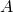and Io eclipsed by Jupiter. The next eclipse then occurs when Earth is at point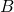, to which the light carrying the information of this eclipse must travel. Sinceis closer to Jupiter than, light takes less time to reach Earth when it is at. This time interval between the successive eclipses of Io seen atandis therefore less than the time interval between the eclipses seen at A and B. By measuring the difference in these time intervals and with appropriate knowledge of the distance between Jupiter and Earth, Roemer calculated that the speed of light was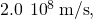which is 33% below the value accepted today.

Roemer’s astronomical method for determining the speed of light. Measurements of Io’s period done with the configurations of parts (a) and (b) differ, because the light path length and associated travel time increase from A to B (a) but decrease fromto(b).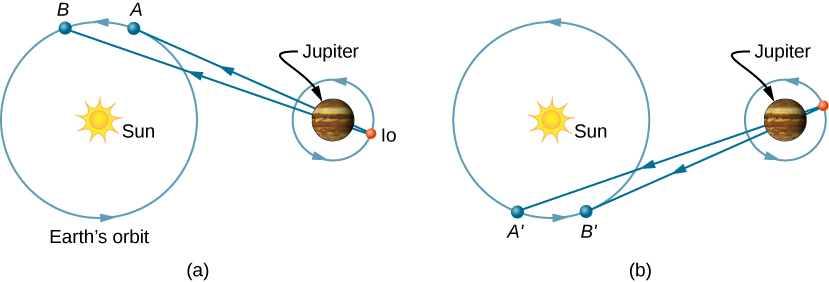The first successful terrestrial measurement of the speed of light was made by Armand Fizeau (1819–1896) in 1849. He placed a toothed wheel that could be rotated very rapidly on one hilltop and a mirror on a second hilltop 8 km away ((Figure)). An intense light source was placed behind the wheel, so that when the wheel rotated, it chopped the light beam into a succession of pulses. The speed of the wheel was then adjusted until no light returned to the observer located behind the wheel. This could only happen if the wheel rotated through an angle corresponding to a displacement of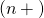teeth, while the pulses traveled down to the mirror and back. Knowing the rotational speed of the wheel, the number of teeth on the wheel, and the distance to the mirror, Fizeau determined the speed of light to be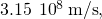which is only 5% too high.

Fizeau’s method for measuring the speed of light. The teeth of the wheel block the reflected light upon return when the wheel is rotated at a rate that matches the light travel time to and from the mirror.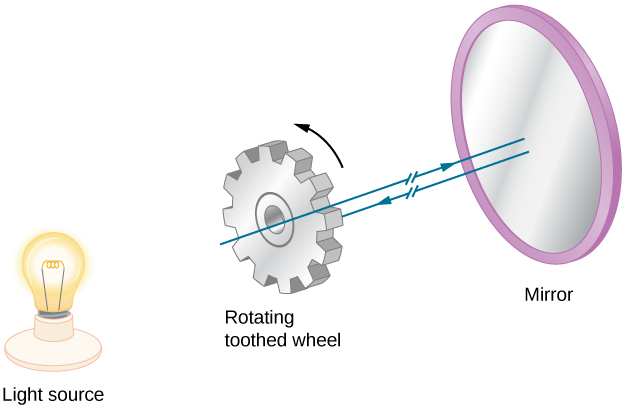The French physicist Jean Bernard Léon Foucault (1819–1868) modified Fizeau’s apparatus by replacing the toothed wheel with a rotating mirror. In 1862, he measured the speed of light to be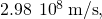which is within 0.6% of the presently accepted value. Albert Michelson (1852–1931) also used Foucault’s method on several occasions to measure the speed of light. His first experiments were performed in 1878; by 1926, he had refined the technique so well that he found c to be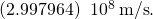Today, the speed of light is known to great precision. In fact, the speed of light in a vacuum c is so important that it is accepted as one of the basic physical quantities and has the value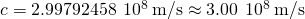where the approximate value of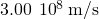is used whenever three-digit accuracy is sufficient.

### Speed of Light in Matter

The speed of light through matter is less than it is in a vacuum, because light interacts with atoms in a material. The speed of light depends strongly on the type of material, since its interaction varies with different atoms, crystal lattices, and other substructures. We can define a constant of a material that describes the speed of light in it, called the index of refraction n: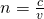where v is the observed speed of light in the material.

Since the speed of light is always less than c in matter and equals c only in a vacuum, the index of refraction is always greater than or equal to one; that is,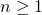. (Figure) gives the indices of refraction for some representative substances. The values are listed for a particular wavelength of light, because they vary slightly with wavelength. (This can have important effects, such as colors separated by a prism, as we will see in Dispersion.) Note that for gases, n is close to 1.0. This seems reasonable, since atoms in gases are widely separated, and light travels at c in the vacuum between atoms. It is common to take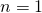for gases unless great precision is needed. Although the speed of light v in a medium varies considerably from its value c in a vacuum, it is still a large speed.

Index of Refraction in Various MediaFor light with a wavelength of 589 nm in a vacuum
Medium n
Gases at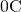, 1 atm
Air 1.000293
Carbon dioxide 1.00045
Hydrogen 1.000139
Oxygen 1.000271
Liquids at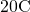Benzene 1.501
Carbon disulfide 1.628
Carbon tetrachloride 1.461
Ethanol 1.361
Glycerine 1.473
Water, fresh 1.333
Solids atDiamond 2.419
Fluorite 1.434
Glass, crown 1.52
Glass, flint 1.66
Ice (at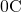1.309
Polystyrene 1.49
Plexiglas 1.51
Quartz, crystalline 1.544
Quartz, fused 1.458
Sodium chloride 1.544
Zircon 1.923

Speed of Light in Jewelry Calculate the speed of light in zircon, a material used in jewelry to imitate diamond.

Strategy We can calculate the speed of light in a material v from the index of refraction n of the material, using the equation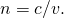Solution Rearranging the equation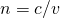for v gives us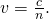The index of refraction for zircon is given as 1.923 in (Figure), and c is given in (Figure). Entering these values in the equation gives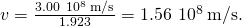Significance This speed is slightly larger than half the speed of light in a vacuum and is still high compared with speeds we normally experience. The only substance listed in (Figure) that has a greater index of refraction than zircon is diamond. We shall see later that the large index of refraction for zircon makes it sparkle more than glass, but less than diamond.

Check Your Understanding(Figure) shows that ethanol and fresh water have very similar indices of refraction. By what percentage do the speeds of light in these liquids differ?

2.1% (to two significant figures)

### The Ray Model of Light

You have already studied some of the wave characteristics of light in the previous chapter on Electromagnetic Waves. In this chapter, we start mainly with the ray characteristics. There are three ways in which light can travel from a source to another location ((Figure)). It can come directly from the source through empty space, such as from the Sun to Earth. Or light can travel through various media, such as air and glass, to the observer. Light can also arrive after being reflected, such as by a mirror. In all of these cases, we can model the path of light as a straight line called a ray.

Three methods for light to travel from a source to another location. (a) Light reaches the upper atmosphere of Earth, traveling through empty space directly from the source. (b) Light can reach a person by traveling through media like air and glass. (c) Light can also reflect from an object like a mirror. In the situations shown here, light interacts with objects large enough that it travels in straight lines, like a ray.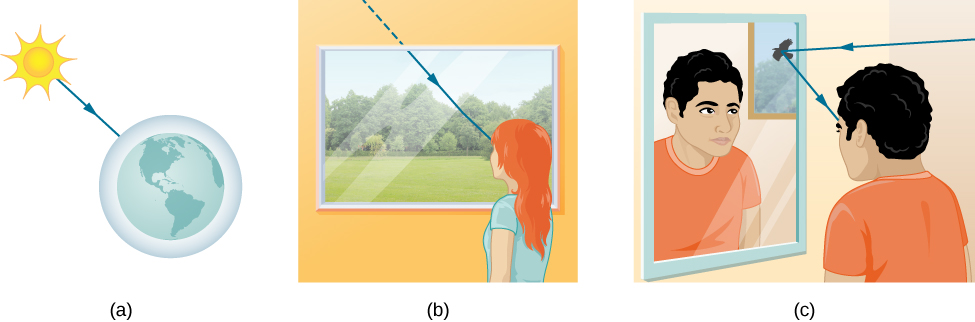Experiments show that when light interacts with an object several times larger than its wavelength, it travels in straight lines and acts like a ray. Its wave characteristics are not pronounced in such situations. Since the wavelength of visible light is less than a micron (a thousandth of a millimeter), it acts like a ray in the many common situations in which it encounters objects larger than a micron. For example, when visible light encounters anything large enough that we can observe it with unaided eyes, such as a coin, it acts like a ray, with generally negligible wave characteristics.

In all of these cases, we can model the path of light as straight lines. Light may change direction when it encounters objects (such as a mirror) or in passing from one material to another (such as in passing from air to glass), but it then continues in a straight line or as a ray. The word “ray” comes from mathematics and here means a straight line that originates at some point. It is acceptable to visualize light rays as laser rays. The ray model of light describes the path of light as straight lines.

Since light moves in straight lines, changing directions when it interacts with materials, its path is described by geometry and simple trigonometry. This part of optics, where the ray aspect of light dominates, is therefore called geometric optics. Two laws govern how light changes direction when it interacts with matter. These are the law of reflection, for situations in which light bounces off matter, and the law of refraction, for situations in which light passes through matter. We will examine more about each of these laws in upcoming sections of this chapter.

### Summary

• The speed of light in a vacuum is.
• The index of refraction of a material is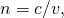where v is the speed of light in a material and c is the speed of light in a vacuum.
• The ray model of light describes the path of light as straight lines. The part of optics dealing with the ray aspect of light is called geometric optics.
• Light can travel in three ways from a source to another location: (1) directly from the source through empty space; (2) through various media; and (3) after being reflected from a mirror.

### Conceptual Questions

Under what conditions can light be modeled like a ray? Like a wave?

Light can be modeled as a ray when devices are large compared to wavelength, and as a wave when devices are comparable or small compared to wavelength.

Why is the index of refraction always greater than or equal to 1?

Does the fact that the light flash from lightning reaches you before its sound prove that the speed of light is extremely large or simply that it is greater than the speed of sound? Discuss how you could use this effect to get an estimate of the speed of light.

This fact simply proves that the speed of light is greater than that of sound. If one knows the distance to the location of the lightning and the speed of sound, one could, in principle, determine the speed of light from the data. In practice, because the speed of light is so great, the data would have to be known to impractically high precision.

Speculate as to what physical process might be responsible for light traveling more slowly in a medium than in a vacuum.

### Problems

What is the speed of light in water? In glycerine?

What is the speed of light in air? In crown glass?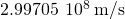;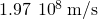Calculate the index of refraction for a medium in which the speed of light is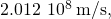and identify the most likely substance based on (Figure).

In what substance in (Figure) is the speed of light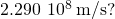ice atThere was a major collision of an asteroid with the Moon in medieval times. It was described by monks at Canterbury Cathedral in England as a red glow on and around the Moon. How long after the asteroid hit the Moon, which is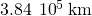away, would the light first arrive on Earth?

Components of some computers communicate with each other through optical fibers having an index of refraction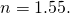What time in nanoseconds is required for a signal to travel 0.200 m through such a fiber?

1.03 ns

Compare the time it takes for light to travel 1000 m on the surface of Earth and in outer space.

How far does light travel underwater during a time interval of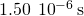?

337 m

### Glossary

geometric optics
part of optics dealing with the ray aspect of light
index of refraction
for a material, the ratio of the speed of light in a vacuum to that in a material
ray
straight line that originates at some point

## LicenseUniversity Physics Volume 3 by cnxuniphysics is licensed under a Creative Commons Attribution 4.0 International License, except where otherwise noted.